# Bernstein problem in mathematical genetics

Jump to: navigation, search

Bernshtein problem

Letbe the simplex inspanned by the canonical basis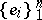. Any set of numbers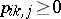(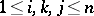) such thatanddefines a stochastic quadratic mappingby the formulas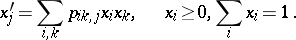This mapping is called Bernstein (or stationary) if. The Bernstein problem is to explicitly describe all such mappings. This problem was posed by S.N. Bernshtein [a1] in order to create a mathematical foundation of population genetics. For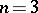, this problem has been solved in [a2]. (For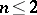the problem is trivial.)

The classical Mendel mechanism of heredity defines a mapping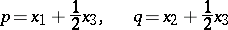(the Hardy–Weinberg laws, cf. [a5]). Biologically,and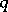are the probabilities of an alternating pair of genes, sayandrespectively, in a population where the individuals may be of genotypes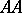,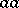and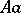. Then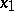,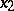,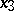are the probabilities of these genotypes in a generation. If the next generation is formed by random mating, then the probabilities turn into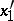,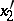,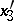. As a result,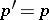,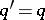and then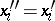(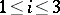), i.e. the Hardy–Weinberg mapping is stationary. Conversely, if fora stationary mappingis such thatand all quadratic forms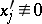, thenis a Hardy–Weinberg mapping (see [a2], [a6]). Thus, the only Mendelian heredity is stationary and such that all offsprings for the parental couple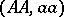are(and, in addition, such that all genotypes are present in the next generation).

For any stochastic quadratic mapping, the linear form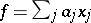is called invariant if. The mappingis called regular if there exists a familyof invariant linear forms such that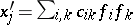for certain constant coefficients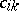. The Hardy–Weinberg mapping is regular. Another interesting example is the quadrille mapping (see [a2], [a6]):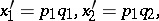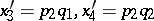where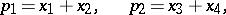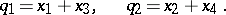For the regular case, the Bernstein problem has been solved in [a6], [a8], [a9]. This is precisely the case when the stationarity is based on a system of genes (see [a7]). The genes correspond to the extremal rays of the cone of non-negative invariant linear forms. After a normalization, these forms are just the probabilities of the genes.

A standard genetical interpretation also requiresto be normal in the sense that:

1) all;

2) for any pair,the quadratic forms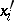and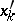are not proportional;

3) there is no pair,such that all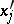are functions ofand of the remaining. Ifis normal together with its restrictions to all invariant faces of, thenis called ultranormal. All stationary ultranormal mappings are regular [a13].

A non-regular stochastic Bernstein mapping appears already for: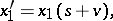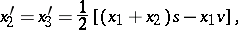where,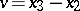. Here, all invariant linear forms are trivial, i.e. proportional to.

For the non-regular case there are some partial results for the Bernstein problem. In particular, the results cover the low dimensions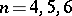(cf. [a3], [a4], [a10]).

In the course of these investigations, Bernstein algebras were introduced as a powerful tool (see [a6], [a8] or Bernstein algebra). The theory of Bernstein algebras was subsequently developed by itself.

In the algebraic context, the Bernstein problem is to explicitly describe those Bernstein algebras which are stochastic with respect to the basis. The latter means that the product of every pair of basis vectors belongs to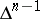.

The Bernstein algebra corresponding to a regular mappingis regular by definition. This class is the most important from the genetics point of view.

Another important tool in the study of the Bernstein problem is a topological structure on the set of essential faces of, the faces such that their intersections with the image ofare non-empty [a11].

See [a12] for a systematic presentation of the results and methods regarding the Bernstein problem up to the middle of the 1980s.